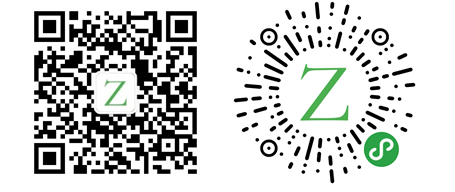# 走进Rust：数据类型

Rust 大约 2589 字

## Scalar Types

### Integer

Signed数值范围：-(2n - 1) 到 2n - 1 - 1，`n``bit`的值。如`i8`可以存储的值范围是：-(27) 到 27 - 1 等于 -128 到 127。

Unsigned数值范围：0 到 2n - 1。如`u8`可以存储的值范围是：0 到 28 - 1 等于 0 到 255。

`isize``usize`类型取决于程序运行的操作系统：`32位`系统是`32bits``64位`系统是`64bits`

Length Signed Unsigned
8-bit i8 u8
16-bit i16 u16
32-bit i32 u32
64-bit i64 u64
128-bit i128 u128
arch isize usize

Number literals Example
Decimal 98_222
Hex 0xff
Octal 0o77
Binary 0b1111_0000
Byte (u8 only) b'A'

`isize``usize`类型的使用场景是：对一些集合进行索引时。

### Floating-Point

`Rust`有两种原子类型的浮点数，`f32``f64`，分别占用`32`比特和`64`比特的空间大小。默认的浮点数类型是`f64`，因为在现代的`CPU``f64`在速度上与`f32`相差无几，但`f64`精度更高。

``````fn main() {
// 加
// 15
let sum = 5 + 10;
println!("sum={}", sum);

// 减
// 91.2
let difference = 95.5 - 4.3;
println!("difference={}", difference);

// 乘
// 120
let product = 4 * 30;
println!("product={}", product);

// 除
// 1.7608695652173911
let quotient = 56.7 / 32.2;
println!("quotient={}", quotient);

// 模
// 3
let remainder = 43 % 5;
println!("remainder={}", remainder);
}``````

### Boolean

`bool`布尔类型主要作用是做流程控制，如`if`表达式。占用`1`个字节的空间大小。`1`字节=`8`比特。

``````fn main() {
let t = true;

let f: bool = false; // 明确指定类型
}``````

### Character

`char`字符类型是`Rust`中最原始字母类型，使用单引号指定。表示重音字母、中日韩的字符、`emoji`表情和零宽度空格。占用`4`个字节空间大小。

``````fn main() {
let c = 'z';
let z = 'ℤ';
let heart_eyed_cat = '😻';

println!("c={}", c);
println!("z={}", z);
println!("heart_eyed_cat={}", heart_eyed_cat);
}``````

## Compound Types

### Tuple

``````fn main() {
let tup = (500, 6.4, 1);
let (x, y, z) = tup;
// x=500, y= 6.4, z=1
println!("x={}, y= {}, z={}", x, y, z);

let x: (i32, f64, u8) = (500, 6.4, 1);
let index1 = x.0;
let index2 = x.1;
let index3 = x.2;
// index1=500, index2= 6.4, index3=1
println!("index1={}, index2= {}, index3={}", index1, index2, index3);
}``````

### Array

``````fn main() {
let months = ["January", "February", "March", "April", "May", "June", "July",
"August", "September", "October", "November", "December"];

let a: [i32; 5] = [1, 2, 3, 4, 5];
let first = a;
let second = a;

// 等价于 let a = [3, 3, 3, 3, 3];
let b = [3; 5];
}``````

————        END        ————

Give me a Star, Thanks:)

https://github.com/fendoudebb